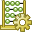# Modifying the CFIE Factor

Activate the combined field integral equation (CFIE) for the model and specify the factor for the linear combination of the magnetic field integral equation (MFIE) and the electric field integral equation (EFIE).

1. On the Solve/Run tab, in the Solution Settings group, click theSolver Settings icon.
2. On the Solver Settings dialog, click the Advanced tab.
3. Under Integral equation settings, select the CFIE factor check box and in the edit field, enter a value for the CFIE factor where 0 < CFIE factor < 1.
Note: The default factor is 0.2. This is a ratio of 20% MFIE to 80% EFIE.
4. Click OK to close the dialog.